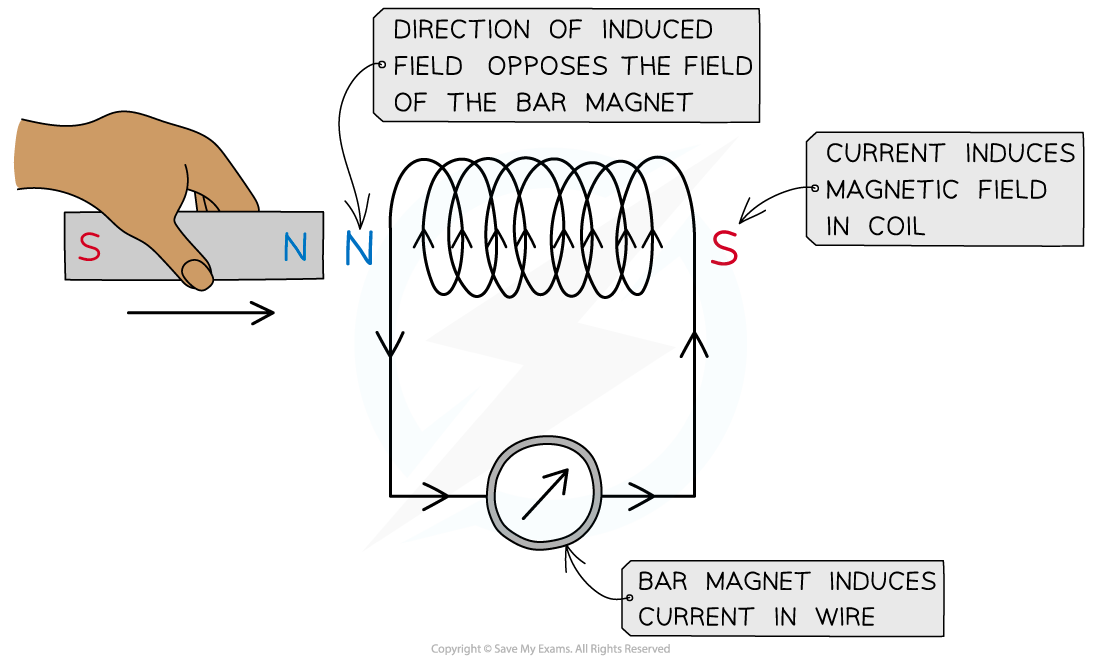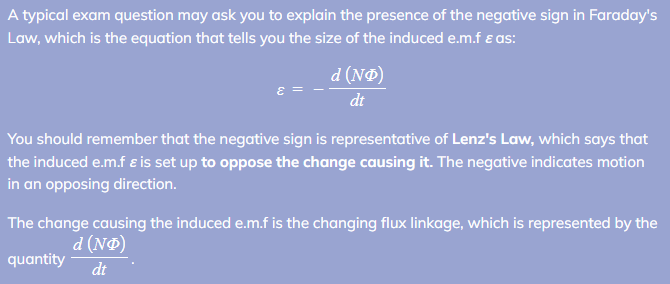# IB DP Physics: HL复习笔记11.1.4 Lenz’s Law

### Lenz's Law

• Lenz’s Law is used to predict the direction of an induced e.m.f in a coil or wire
• Lenz's Law is summarised below:

The induced e.m.f is set up in a direction to produce effects that oppose the change causing it

#### Experimental Evidence for Lenz’s Law

• To verify Lenz’s Law, the only apparatus needed is:
• A bar magnet
• A coil of wire
• A sensitive ammeter
• Note, a cell is not requiredLenz’s law can be verified using a coil connected in series with a sensitive ammeter and a bar magnet

• A known pole (either north or south) of a bar magnet is pushed into the coil
• This induces an e.m.f in the coil
• The induced e.m.f drives a current (because it is a complete circuit)
• Lenz's Law dictates:
• The direction of the e.m.f, and hence the current, must be set up to oppose the incoming magnet
• Since a north pole approaches the coil face, the e.m.f must be set up to create an induced north pole
• This is because two north poles will repel each other
• The direction of the current is therefore as shown in the image above
• The direction of current can be verified using the right hand grip rule
• Fingers curl around the coil in the direction of current and the thumb points along the direction of the flux lines, from north to south
• Therefore, the current flows in an anti-clockwise direction in the image shown, in order to induce a north pole opposing the incoming magnet
• Reversing the magnet direction would give an opposite deflection on the voltmeter
• Lenz's Law now predicts a south pole induced at the coil entrance
• This would attract the north pole attempting to leave
• Therefore, the induced e.m.f always produces effects to oppose the changes causing it
• Lenz's Law is a direct consequence of the principle of conservation of energy
• Electromagnetic effects will not create electrical energy out of nothing
• In order to induce and sustain an e.m.f, for instance, work must be done in order to overcome the repulsive effect due to Lenz's Law

#### Exam Tip